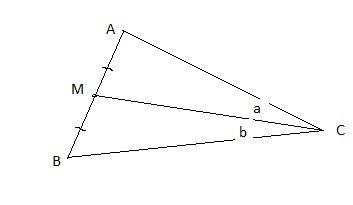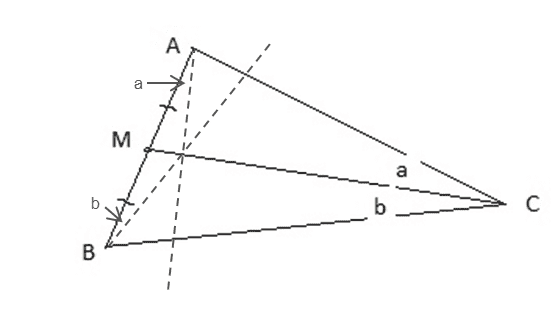# Geometrical Proof

## Homework Statement

Consider an triangle ABC with M as the middle point of the side AB.
On the straight line through AB you put the angle ∠ ACM at A and the angle ∠ MCB at B. Now you have two new lines. The new lines should be on the same side of AB as C.
Proof that the intersection point of the two new lines is located on the line through CM.

## The Attempt at a Solution

I wanted to use Cevas Theorem but I could not use it :(

I hope you can give me some advice.

You've generated some similar triangles there which should help, if you work out the scaling. Note that |AM| = |BM|

Mark44
Mentor

## Homework Statement

Consider an triangle ABC with M as the middle point of the side AB.
On the straight line through AB you put the angle ∠ ACM at A and the angle ∠ MCB at B. Now you have two new lines.
I don't understand. From your explanation you would have two new angles. In my drawing below I have labelled ∠ ACM as a and ∠ MCB as b.
franceboy said:
The new lines should be on the same side of AB as C.
Proof that the intersection point of the two new lines is located on the line through CM.
???
franceboy said:

## The Attempt at a Solution

I wanted to use Cevas Theorem but I could not use it :(
What is Ceva's Theorem?
franceboy said:
I hope you can give me some advice.Hi Mark, this is the construction as I understand it:Mark44
Mentor
Sorry thai i did not add a sketch but a GeoGebra sketch was not accepted as file.
Joffan your sketch is correct. I used the symmetry so that I " only" need to proof
BX / XC * CY / YA = 1
I determined all the angles and I found some similarities but they did not help to solve the problem.
Is Ceva' s theorem the right idea to solve the problem?

Maybe you could use Ceva's theorem - it seems a little overpowered.

I would proceed by marking X as the intersection of the new line from A with CM and Y as the intersection of the new line from B with CM. Then show that |MX| = |MY| and thus that X == Y